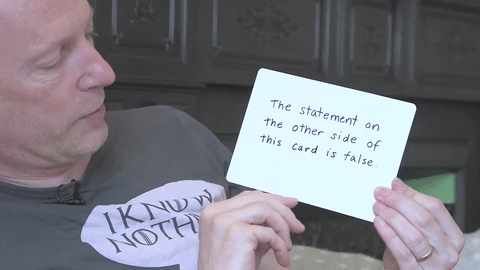# Numberphile looks at mathematics' undecidable statements

The average person probably assumes that mathematics is a complete system in which all mathematical statements can be proved or disproved. The fine folks at Numberphile are ready to disabuse folks of this notion with a nice overview of Gödel's Incompleteness Theorem.Here's a shorter and somewhat simpler explainer:

Gödel's Incompleteness Theorem (YouTube / Numberphile)